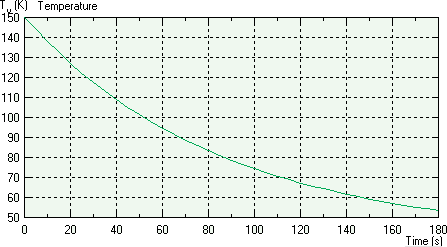QuickField

A new approach to field modelling
 Language: Global English Deutch Espanol Francais Italiano Danmark Ceske Chinese Pycckuü

Main >> Applications >> Sample problems >> Temperature response of a suddenly cooled wire

# Temperature response of a suddenly cooled wire

Determine the temperature response of a copper wire of diameter d, originally at temperature T0, when suddenly immersed in air at temperature Tair. The convection coefficient between the wire and the air is α.

Problem Type:
Plane problem of heat transfer.

Geometry:

d = 0.1 cm.

Given:
Copper initial temperature T0 = 150°C,
thermal conductivity λ = 391 W/m·K,
specific heat C = 383 J/kg·K,
volume mass density ρ = 8930 kg/m3;
Air temperature Tair = 40°C,
convection coefficient α = 10 W/K·m2.

Problem:
Determine the temperature in the wire.

Solution:
A time step of 1 s is used to run numerical analysis.
Analytical solution*: (T - Tair) / (T0 - Tair) = exp(-0.0117·t)

Results:

Temperature in a wire:Temperature, °C Time QuickField Reference* 30 s 117.5 117.4 60 s 94.6 94.5 120 s 67.1 67.0

* Reference: Frank Kreith, Raj M. Manglik, Mark S. Bohn Principles of Heat Transfer, Cengage Learning, Jun 10, 2010 - Technology & Engineering - 696 pages, Page 119, Example 2-10.

• Video: